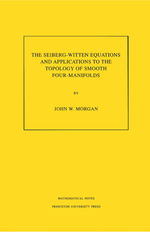# The Seiberg-Witten Equations and Applications to the Topology of Smooth Four-Manifolds. (MN-44)

John W. Morgan
Pages: 130
https://www.jstor.org/stable/j.ctt7ztfpc

1. Front Matter
(pp. i-iv)
(pp. v-viii)
3. Chapter 1 Introduction
(pp. 1-4)

Beginning with the groundbreaking work of Donaldson in about 1980 it became clear that gauge-theoretic invariants of principal bundles and connections were an important tool in the study of smooth four-dimensional manifolds. Donaldson showed the importance of the moduli space of antiself-dual connections. The next fifteen years saw an explosion of work in this area leading to computations of Donaldson polynomial invariants for a wide class of four-dimensional manifolds, especially algebraic surfaces. These computations yielded many powerful topological consequences including, for example, the diffeomorphism classification of elliptic surfaces. For some of the results obtained by using these techniques see ...

4. Chapter 2 Clifford Algebras and Spin Groups
(pp. 5-22)

For anyn> 2 the orthogonal groupSO(n) has fundamental group Z/2Z and hence has a universal covering group calledSpin(n) which is a non-trivial double covering. Thete is a beautiful algebraic construction which yields the Spin groups (and much more as well). This is the subject of Clifford algebras.

An example. Befote delving into the complexities of Clifford algebras in general, let us consider a simple example. Consider the unit sphereS3inside the quaternion algebra H. Multiplication of quaternions induces a group structure onS3. Let us consider the action of this group on H by conjugation

s3...

5. Chapter 3 Spin Bundles and the Dirac Operator
(pp. 23-54)

Spin Bundles. Fix a real vector spaceVwith a positive definite inner product. We suppose throughout this chapter that the dimension ofVis at least 2. Suppose thatPXis a principalSO(V) bundle. We wish to understand when this bundle lifts to a principalSpin(V) bundle; that is to say, when there is a principalSpin(V) bundle$\tilde{P}\to X$whose quotient by the center ±1 ofSpin(V) is isomorphic as anSO(V) bundle toP. This is of course, a standard problem in algebraic topology or obstruction theory whose solution is well-known.

Lemma 3.1.1The bundle...

6. Chapter 4 The Seiberg-Witten Moduli Space
(pp. 55-68)

We are now in a position to write down the Seiberg-Witten Equations for a closed, oriented riemannian four-manifoldX. The equations require aSpincstructure$\tilde{P}$for the orthogonal frame bundlePXof the tangent bundle ofX. We denote by$\script{L}$the determinant line bundle of$\tilde{P}$. From now on we denote the complex plus and minus spin bundles associated to$\tilde{P}$byS±($\tilde{P}$). Recall thatS±($\tilde{P}$) has a hermitian inner product and hence so does$\script{L}$which is identified with the determinant line bundle of bothS±($\tilde{P}$). The Seiberg-Witten...

7. Chapter 5 Curvature Identities and Bounds
(pp. 69-86)

The main result in this chapter is the compactness of the moduli space of solutions to the Seiberg-Witten equations. In fact, it is a very strong form of compactness: for any metric onXthere are only finitely many non-empty moduli spaces and each of these is compact. (Actually, there is a hidden assumption here, we are dealing only with moduli spaces whose formal dimensions are non-negative. Of course, if we choose a generic perturbation of the equations, then the only non-empty moduli spaces will have formal dimensions which are non-negative.) This compactness result is a consequence of two phenomena....

8. Chapter 6 The Seiberg-Witten Invariant
(pp. 87-108)

In general, there is no reason to expect that the space of solutions to the Seiberg-Witten equations form a smooth manifold. The best that one can hope for is that generically they do. In theSU(2)-ASD connection context one uses the metric as a parameter and shows that for a generic metric the ASD moduli space is smooth, see Section 3.2 of Chapter III of . In this context there is no known generic metrics theorem. Instead we use a different family of perturbations. We perturb the curvature equation by adding a purely imaginary self-dual two-form.

Theorem 6.1.1Suppose that...

9. Chapter 7 Invariants of Kähler Surfaces
(pp. 109-126)

In order to explicitly evaluate the Seiberg-Witten invariant of a compact Kähler surface we begin by rewriting the Seiberg-Witten equations in terms of the complex geometry. From this description it will be a fairly easy matter to completely solve the equations over such a four-manifold in terms of standard holomorphic objects.

In this section we shall give an explicit description of the Seiberg-Witten moduli space when the base riemannian four-manifold is a compact Kähler surface. Recall from Chapter 3 that an orthogonal almost complex structureJ:TXTXon a riemannian four-manifold induces aSpincstructure${{\tilde{P}}_{J}}\to X$whose determinant...

10. Bibliography
(pp. 127-129)